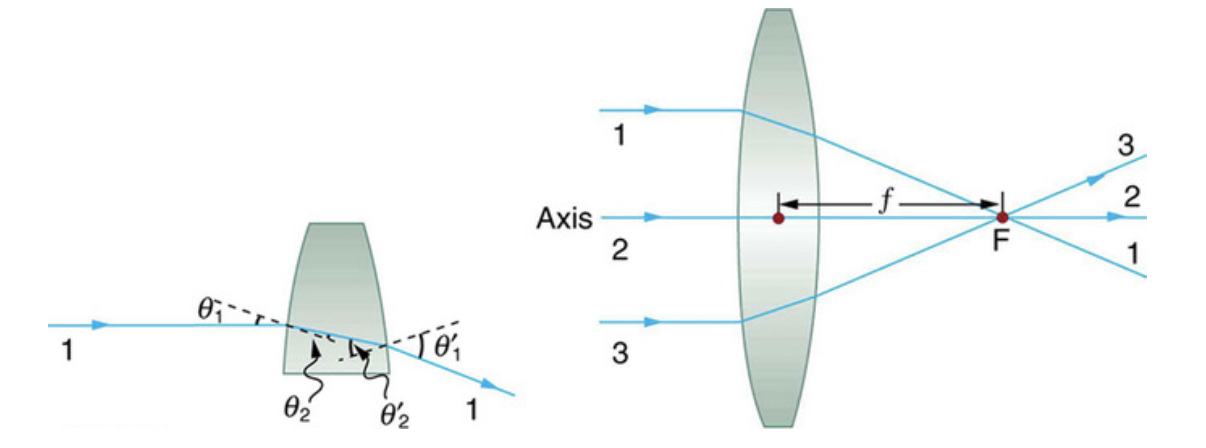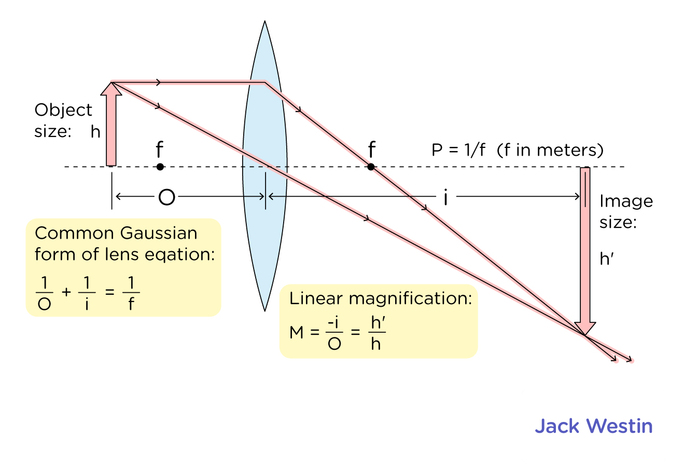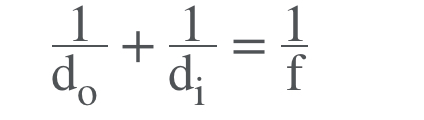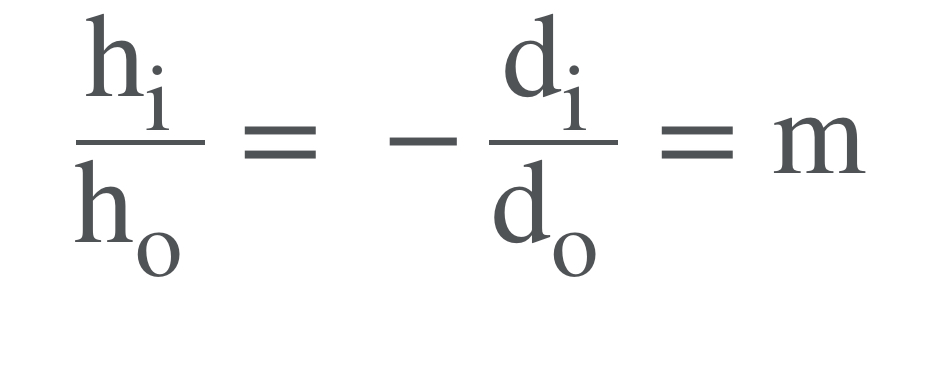Having trouble writing your personal statement?

### Thin Lenses

Topic: Geometrical Optics

A thin lens allows rays to refract but does not allow properties such as dispersion and aberrations.

An ideal thin lens has two refracting surfaces but the lens is thin enough to assume that light rays bend only once. Another way of saying this is that the lens thickness is much much smaller than the focal length of the lens. A thin symmetrical lens has two focal points, one on either side and both at the same distance from the lens. Another important characteristic of a thin lens is that light rays through its center are deflected by a negligible amount. The treatment of a lens as a thin lens is known as the “thin lens approximation”.Converging lens: Parallel light rays entering a converging lens from the right cross at its focal point on the left (a).

Diverging lens: Parallel light rays entering a diverging lens from the right seem to come from the focal point on the right (b).Thin lens equation relates the object distance (do), image distance (di), and focal length (f). The magnification m of an image is the ratio between the image and object height (hi/ho). Like mirrors, the image formed by refraction may be real or virtual. A real image occurs when light rays actually intersect at the image and is inverted, or upside down. A virtual image happens when light rays do not meet at the image. Instead, you “see” the image because your eye projects light rays backwards. A virtual image is right side up (upright).The lens strength can be measured in diopters and can be found by using the equation P= 1/f where f is the focal length and P is diopters of the lens.Practice Questions

Hyperopia and convex lenses

Converging and diverging lenses in a lab

A mirror in an operating room

MCAT Official Prep (AAMC)

Physics Online Flashcards Question 12

Physics Question Pack Passage 8 Question 51

Physics Question Pack Question 118

Practice Exam 1 C/P Section Question 11

Practice Exam 1 C/P Section Question 45

Section Bank C/P Section Passage 2 Question 11

Practice Exam 2 C/P Section Passage 10 Question 55

Key Points

• A thin lens is defined as one with a thickness that allows rays to refract, but does not allow dispersion and aberrations. An ideal thin lens has two refracting surfaces but the lens is thin enough to assume that light rays bend only once.

• The thin lens equation: 1/do + 1/di = 1/f

Key Terms

Focal point: halfway between the mirror and the center of curvature on the principal axis.

Magnification: of an image (m) is the ratio between the image and object height (hi/ho).

Billing Information